# Link Prediction with GDSL and scikit-learn

 Important - page not maintained This page is no longer being maintained and its content may be out of date. For the latest guidance, please visit the Neo4j Graph Data Science Library .
Goals
In this guide, we will learn how to solve a link prediction problem using a scikit-learn binary classifier and the Graph Data Science Library.
Prerequisites
Please have Neo4j (version 4.0 or later) and the Graph Data Science Library downloaded and installed. You will also need to install the Python scikit-learn library.

Intermediate

Link Prediction techniques are used to predict future or missing links in graphs. In this guide we’re going to use these techniques to predict future co-authorships using scikit-learn and link prediction algorithms from the Graph Data Science Library.

 The code examples used in this guide can be found in the neo4j-examples/link-prediction GitHub repository. For background reading on link prediction, see the Link Prediction guide.

## Citation GraphIn this guide, we’re going to use data from the DBLP Citation Network, which includes citation data from various academic sources. The full dataset is very large, but we’re going to use a subset that contains data from a few Software Development Conferences.

A screenshot of the available datasets is shown below: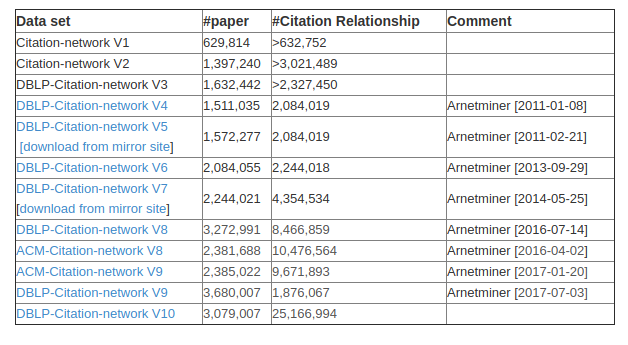Figure 1. Citation Networks

You can find instructions for importing the data in the project repository. The following diagram shows what the data looks like once we’ve imported into Neo4j:Figure 2. Diagram showing Citation Network in Neo4j

### Building a co-author graph

The dataset doesn’t contain relationships between authors describing their collaborations, but we can infer them based on finding articles authored by multiple people. The code below creates a `CO_AUTHOR` relationship between authors that have collaborated on at least one article:

``````from neo4j import GraphDatabase

query = """
CALL apoc.periodic.iterate(
"MATCH (a1)<-[:AUTHOR]-(paper)-[:AUTHOR]->(a2:Author)
WITH a1, a2, paper
ORDER BY a1, paper.year
RETURN a1, a2, collect(paper).year AS year, count(*) AS collaborations",
"MERGE (a1)-[coauthor:CO_AUTHOR {year: year}]-(a2)
SET coauthor.collaborations = collaborations",
{batchSize: 100})
"""

with driver.session(database="neo4j") as session:
result = session.run(query)``````

We create only one `CO_AUTHOR` relationship between authors that have collaborated, even if they’ve collaborated on multiple articles. We create a couple of properties on these relationships:

• a `year` property that indicates the publication year of the first article on which the authors collaborated

• a `collaborations` property that indicates how many articles on which the authors have collaboratedFigure 3. The co-authors graph

## Train and test datasets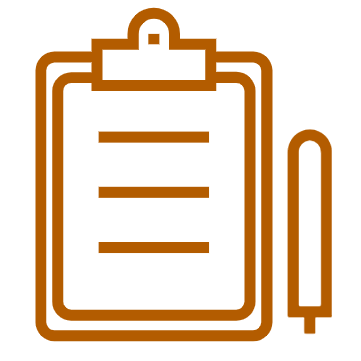To avoid data leakage, we need to split our graph into training and test sub graphs. We are lucky that our citation graph contains time information that we can split on. We will create training and test graphs by splitting the data on a particular year.

But which year should we split on? Let’s have a look at the distribution of the first year that co-authors collaborated:

``````import pandas as pd
import matplotlib
import matplotlib.pyplot as plt

plt.style.use('fivethirtyeight')
pd.set_option('display.float_format', lambda x: '%.3f' % x)

query = """
MATCH p=()-[r:CO_AUTHOR]->()
WITH r.year AS year, count(*) AS count
ORDER BY year
"""

with driver.session(database="neo4j") as session:
result = session.run(query)
by_year = pd.DataFrame([dict(record) for record in result])

ax = by_year.plot(kind='bar', x='year', y='count', legend=None, figsize=(15,8))
ax.xaxis.set_label_text("")
plt.tight_layout()

plt.show()``````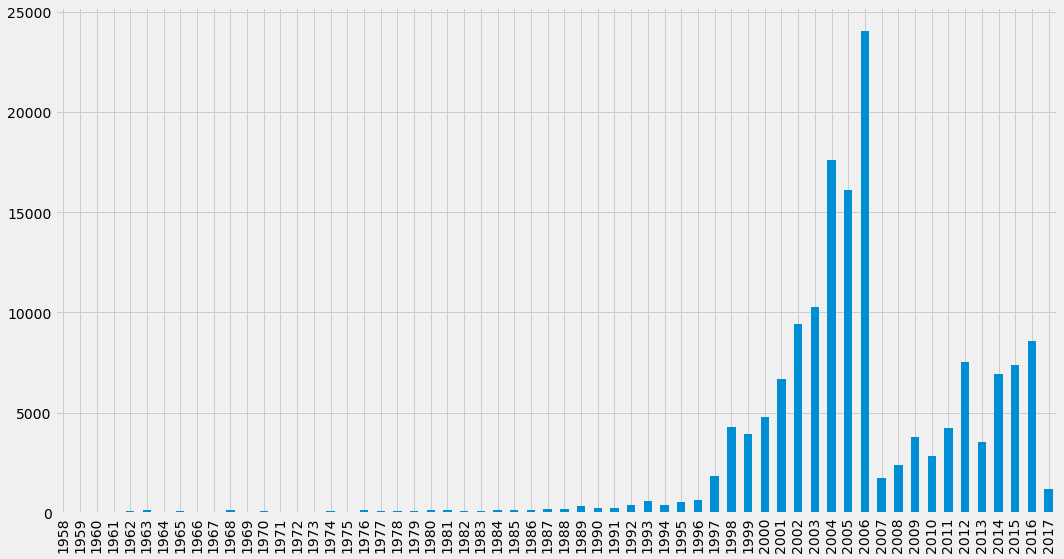Figure 4. Chart showing distribution of year of collaboration

It looks like 2006 would act as a good year on which to split the data, because it will give us a reasonable amount of data for each of our sub graphs. We’ll take all the co-authorships from 2005 and earlier as our training graph, and everything from 2006 onwards as the test graph.

Let’s create explicit `CO_AUTHOR_EARLY` and `CO_AUTHOR_LATE` relationships in our graph based on that year. The following code will create these relationships for us:

``````query = """
MATCH (a)-[r:CO_AUTHOR]->(b)
where r.year < 2006
MERGE (a)-[:CO_AUTHOR_EARLY {year: r.year}]-(b);
"""

with driver.session(database="neo4j") as session:
display(session.run(query).consume().counters)

query = """
MATCH (a)-[r:CO_AUTHOR]->(b)
where r.year >= 2006
MERGE (a)-[:CO_AUTHOR_LATE {year: r.year}]-(b);
"""

with driver.session(database="neo4j") as session:
display(session.run(query).consume().counters)``````

This split leaves us with 81,096 relationships in the early graph, and 74,128 in the late one. This is a split of 52–48. That’s a higher percentage of values than we’d usually have in our test graph, but it should be ok. The relationships in these sub graphs will act as the positive examples in our train and test sets, but we need some negative examples as well. The negative examples are needed so that our model can learn to distinguish nodes that should have a link between them and nodes that should not.

As is often the case in link prediction problems, there are a lot more negative examples than positive ones. Instead of using almost all possible pairs, we’ll use pairs of nodes that are between 2 and 3 hops away from each other. This will give us a much more manageable amount of data to work with.

We can generate these pairs by running the following code:

``````with driver.session(database="neo4j") as session:
result = session.run("""
MATCH (author:Author)-[:CO_AUTHOR_EARLY]->(other:Author)
RETURN id(author) AS node1, id(other) AS node2, 1 AS label""")
train_existing_links = pd.DataFrame([dict(record) for record in result])

result = session.run("""
MATCH (author:Author)
WHERE (author)-[:CO_AUTHOR_EARLY]-()
MATCH (author)-[:CO_AUTHOR_EARLY*2..3]-(other)
WHERE not((author)-[:CO_AUTHOR_EARLY]-(other))
RETURN id(author) AS node1, id(other) AS node2, 0 AS label""")
train_missing_links = pd.DataFrame([dict(record) for record in result])

Let’s combine the `train_existing_links` and `train_missing_links` DataFrames and check how many positive and negative examples we have:

``````training_df = train_missing_links.append(train_existing_links, ignore_index=True)
training_df['label'] = training_df['label'].astype('category')

count_class_0, count_class_1 = training_df.label.value_counts()
print(f"Negative examples: {count_class_0}")
print(f"Positive examples: {count_class_1}")``````
``````Negative examples: 973019
Positive examples: 81096``````

We have more than 10 times as many negative examples as positive ones. So we still have a big class imbalance. To solve this issue we can either up sample the positive examples or down sample the negative examples.

 The advantages and disadvantages of each approach are described in Resampling strategies for imbalanced datasets.

We’re going to go with the downsampling approach. We can downsample the negative examples using the following code:

``````df_class_0 = training_df[training_df['label'] == 0]
df_class_1 = training_df[training_df['label'] == 1]

df_class_0_under = df_class_0.sample(count_class_1)
df_train_under = pd.concat([df_class_0_under, df_class_1], axis=0)

print('Random downsampling:')
print(df_train_under.label.value_counts())``````
``````Random downsampling:
1    81096
0    81096
Name: label, dtype: int64``````

We’ll now do the same thing for the test data, using the following code:

``````with driver.session(database="neo4j") as session:
result = session.run("""
MATCH (author:Author)-[:CO_AUTHOR_LATE]->(other:Author)
RETURN id(author) AS node1, id(other) AS node2, 1 AS label""")
test_existing_links = pd.DataFrame([dict(record) for record in result])

result = session.run("""
MATCH (author:Author)
WHERE (author)-[:CO_AUTHOR_LATE]-()
MATCH (author)-[:CO_AUTHOR_LATE*2..3]-(other)
WHERE not((author)-[:CO_AUTHOR_LATE]-(other))
RETURN id(author) AS node1, id(other) AS node2, 0 AS label""")
test_missing_links = pd.DataFrame([dict(record) for record in result])

test_df['label'] = test_df['label'].astype('category')

count_class_0, count_class_1 = test_df.label.value_counts()
print(f"Negative examples: {count_class_0}")
print(f"Positive examples: {count_class_1}")

df_class_0 = test_df[test_df['label'] == 0]
df_class_1 = test_df[test_df['label'] == 1]

df_class_0_under = df_class_0.sample(count_class_1)
df_test_under = pd.concat([df_class_0_under, df_class_1], axis=0)

print('Random downsampling:')
print(df_test_under.label.value_counts())``````
``````Negative examples: 1265118
Positive examples: 74128

Random downsampling:
1    74128
0    74128
Name: label, dtype: int64``````

Before we move on, let’s have a look at the contents of our train and test DataFrames:

``df_train_under.sample(5, random_state=42)``
Table 1. Train
node1 node2 label

101053

101054

1

35945

77989

1

2657

2658

1

213982

228457

0

95994

71626

0

``df_test_under.sample(5, random_state=42)``
Table 2. Test
node1 node2 label

101053

101054

1

35945

77989

1

2657

2658

1

213982

228457

0

95994

71626

0

## Feature Engineering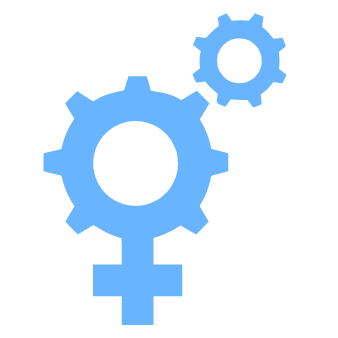Now it’s time to engineer some features which we’ll use to train our model. We’re going to create three types of features:

features from running the link prediction algorithms

Triangles and Clustering Coefficient

features from running the triangles and clustering coefficient algorithms

Community Detection

features from running the Louvain and Label Propagation algorithms

We’ll start by creating some features using link prediction functions. We can do this by applying the following function over the test and train DataFrames:

``````def apply_graphy_features(data, rel_type):
query = """
UNWIND \$pairs AS pair
MATCH (p1) WHERE id(p1) = pair.node1
MATCH (p2) WHERE id(p2) = pair.node2
RETURN pair.node1 AS node1,
pair.node2 AS node2,
relationshipQuery: \$relType}) AS cn,
relationshipQuery: \$relType}) AS pa,
relationshipQuery: \$relType}) AS tn
"""
pairs = [{"node1": node1, "node2": node2}  for node1,node2 in data[["node1", "node2"]].values.tolist()]

with driver.session(database="neo4j") as session:
result = session.run(query, {"pairs": pairs, "relType": rel_type})
features = pd.DataFrame([dict(record) for record in result])
return pd.merge(data, features, on = ["node1", "node2"])

df_train_under = apply_graphy_features(df_train_under, "CO_AUTHOR_EARLY")
df_test_under = apply_graphy_features(df_test_under, "CO_AUTHOR")``````

This function adds three columns to the train and test DataFrames:

• `cn` - common neighbors score

• `pa` - preferential attachment score

• `tn` - total neighbors score

For the training DataFrame we compute these metrics based only on the early graph, whereas for the test DataFrame we’ll compute them across the whole graph.

### Triangles and Clustering Coefficient

Now we’re going to add some new features that are generated using the triangles and clustering coefficient algorithms, where:

• Triangle count computer the number of triangles that each node forms.

• Clustering coefficient computes the likelihood that its neighbors are also connected.

We can compute these metrics for the nodes by running the following code:

Triangles
``````query = """
CALL gds.triangleCount.write({
nodeProjection: 'Author',
relationshipProjection: {
CO_AUTHOR_EARLY: {
type: 'CO_AUTHOR_EARLY',
orientation: 'UNDIRECTED'
}
},
writeProperty: 'trianglesTrain'
});
"""

with driver.session(database="neo4j") as session:
result = session.run(query)

query = """
CALL gds.triangleCount.write({
nodeProjection: 'Author',
relationshipProjection: {
CO_AUTHOR: {
type: 'CO_AUTHOR',
orientation: 'UNDIRECTED'
}
},
writeProperty: 'trianglesTest'
});
"""

with driver.session(database="neo4j") as session:
result = session.run(query)``````
Clustering Coefficient
``````query = """
CALL gds.localClusteringCoefficient.write({
nodeProjection: 'Author',
relationshipProjection: {
CO_AUTHOR_EARLY: {
type: 'CO_AUTHOR',
orientation: 'UNDIRECTED'
}
},
writeProperty: 'coefficientTrain'
});
"""

with driver.session(database="neo4j") as session:
result = session.run(query)

query = """
CALL gds.localClusteringCoefficient.write({
nodeProjection: 'Author',
relationshipProjection: {
CO_AUTHOR_EARLY: {
type: 'CO_AUTHOR',
orientation: 'UNDIRECTED'
}
},
writeProperty: 'coefficientTest'
});
"""

with driver.session(database="neo4j") as session:
result = session.run(query)``````

And we can add the metrics to our test and train DataFrames by running the following code:

``````def apply_triangles_features(data, triangles_prop, coefficient_prop):
query = """
UNWIND \$pairs AS pair
MATCH (p1) WHERE id(p1) = pair.node1
MATCH (p2) WHERE id(p2) = pair.node2
RETURN pair.node1 AS node1,
pair.node2 AS node2,
apoc.coll.min([p1[\$trianglesProp], p2[\$trianglesProp]]) AS minTriangles,
apoc.coll.max([p1[\$trianglesProp], p2[\$trianglesProp]]) AS maxTriangles,
apoc.coll.min([p1[\$coefficientProp], p2[\$coefficientProp]]) AS minCoefficient,
apoc.coll.max([p1[\$coefficientProp], p2[\$coefficientProp]]) AS maxCoefficient
"""
pairs = [{"node1": node1, "node2": node2}  for node1,node2 in data[["node1", "node2"]].values.tolist()]
params = {
"pairs": pairs,
"trianglesProp": triangles_prop,
"coefficientProp": coefficient_prop
}

with driver.session(database="neo4j") as session:
result = session.run(query, params)
features = pd.DataFrame([dict(record) for record in result])

return pd.merge(data, features, on = ["node1", "node2"])

df_train_under = apply_triangles_features(df_train_under, "trianglesTrain", "coefficientTrain")
df_test_under = apply_triangles_features(df_test_under, "trianglesTest", "coefficientTest")``````
 These measures are different than the ones we’ve used so far, because rather than being computed based on the pair of nodes, they are node specific measures. We can’t simply add these values to our DataFrame as `node1Triangles` or `node1Coeff` because we have no guarantee over the order of nodes in the pair. We need to come up with an approach that is agnostic of the order We can do this by taking the average of the values, the product of the values, or by computing the minimum and maximum value, as we do here.

### Community Detection

Finally we’ll add features based on community detection algorithms. Community detection algorithms evaluate how a group is clustered or partitioned. Nodes are considered more similar to nodes that fall in their community than to nodes in other communities.

We’ll run two community detection algorithms over the train and test sub graphs - Label Propagation and Louvain. First, Label Propagation:

Label Propagation
``````query = """
CALL gds.labelPropagation.write({
nodeProjection: "Author",
relationshipProjection: {
CO_AUTHOR_EARLY: {
type: 'CO_AUTHOR_EARLY',
orientation: 'UNDIRECTED'
}
},
writeProperty: "partitionTrain"
});
"""

with driver.session(database="neo4j") as session:
result = session.run(query)

query = """
CALL gds.labelPropagation.write({
nodeProjection: "Author",
relationshipProjection: {
CO_AUTHOR: {
type: 'CO_AUTHOR',
orientation: 'UNDIRECTED'
}
},
writeProperty: "partitionTest"
});
"""

with driver.session(database="neo4j") as session:
result = session.run(query)``````

And now Louvain. The Louvain algorithm returns intermediate communities, which are useful for finding fine grained communities that exist in a graph. We’ll add a property to each node containing the community revealed on the first iteration of the algorithm:

Louvain
``````query = """
CALL gds.louvain.stream({
nodeProjection: 'Author',
relationshipProjection: {
CO_AUTHOR_EARLY: {
type: 'CO_AUTHOR_EARLY',
orientation: 'UNDIRECTED'
}
},
includeIntermediateCommunities: true
})
YIELD nodeId, communityId, intermediateCommunityIds
WITH gds.util.asNode(nodeId) AS node, intermediateCommunityIds AS smallestCommunity
SET node.louvainTrain = smallestCommunity;
"""

with driver.session(database="neo4j") as session:
display(session.run(query).consume().counters)

query = """
CALL gds.louvain.stream({
nodeProjection: 'Author',
relationshipProjection: {
CO_AUTHOR: {
type: 'CO_AUTHOR',
orientation: 'UNDIRECTED'
}
},
includeIntermediateCommunities: true
})
YIELD nodeId, communityId, intermediateCommunityIds
WITH gds.util.asNode(nodeId) AS node, intermediateCommunityIds AS smallestCommunity
SET node.louvainTest = smallestCommunity;
"""

with driver.session(database="neo4j") as session:
display(session.run(query).consume().counters)``````

And we can check whether a pair of nodes are in the same community and add the result to our test and train DataFrames by running the following code:

``````def apply_community_features(data, partition_prop, louvain_prop):
query = """
UNWIND \$pairs AS pair
MATCH (p1) WHERE id(p1) = pair.node1
MATCH (p2) WHERE id(p2) = pair.node2
RETURN pair.node1 AS node1,
pair.node2 AS node2,
"""
pairs = [{"node1": node1, "node2": node2}  for node1,node2 in data[["node1", "node2"]].values.tolist()]
params = {
"pairs": pairs,
"partitionProp": partition_prop,
"louvainProp": louvain_prop
}

with driver.session(database="neo4j") as session:
result = session.run(query, params)
features = pd.DataFrame([dict(record) for record in result])

return pd.merge(data, features, on = ["node1", "node2"])

df_train_under = apply_community_features(df_train_under, "partitionTrain", "louvainTrain")
df_test_under = apply_community_features(df_test_under, "partitionTest", "louvainTest")``````

We’ve now added all of the features, so let’s have a look at the contents of our Train and Test DataFrames:

``df_train_under.drop(columns=["node1", "node2"]).sample(5, random_state=42)``
Table 3. Train DataFrame
cn maxCoefficient maxTriangles minCoefficient minTriangles pa sl sp tn label

4

1

10

1

10

25

1

1

6

1

2

1

3

0.333333

2

12

1

1

5

1

2

1

3

1

3

9

1

1

4

1

0

1

10

1

3

15

0

1

8

0

0

1

5

0.833333

1

8

0

1

6

0

``df_test_under.drop(columns=["node1", "node2"]).sample(5, random_state=42)``
Table 4. Test DataFrame
cn maxCoefficient maxTriangles minCoefficient minTriangles pa sl sp tn label

0

1

28

0.866667

14

48

0

0

14

0

3

0.0689076

38

0.0584795

8

665

0

0

51

1

1

0.333333

1

0

0

6

1

1

4

1

4

0.377778

27

0.152047

18

190

0

0

25

1

2

0.666667

2

0.3

1

15

1

1

6

1

## Model Selection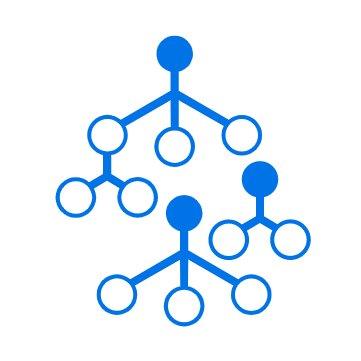Now that we’ve generated all our features, it’s time to create our classifier. We’re going to create a random forest.

This method is well suited as our data set will be comprised of a mix of strong and weak features. While the weak features will sometimes be helpful, the random forest method will ensure we don’t create a model that over fits our training data.

We can create this model with the following code:

``````from sklearn.ensemble import RandomForestClassifier

classifier = RandomForestClassifier(n_estimators=30, max_depth=10, random_state=0)``````

## Model Training

Now let’s build a model based on all these features. We can train the random forest model against the train set by running the following code:

``````columns = [
"cn", "pa", "tn", # graph features
"minTriangles", "maxTriangles", "minCoefficient", "maxCoefficient", # triangle features
"sp", "sl" # community features
]

X = df_train_under[columns]
y = df_train_under["label"]
classifier.fit(X, y)``````

## Model EvaluationWe’re going to evaluate the quality of our model by computing its accuracy, precision, and recall. The diagram below, taken from the O’Reilly Graph Algorithms Book, explains how each of these metrics are computed.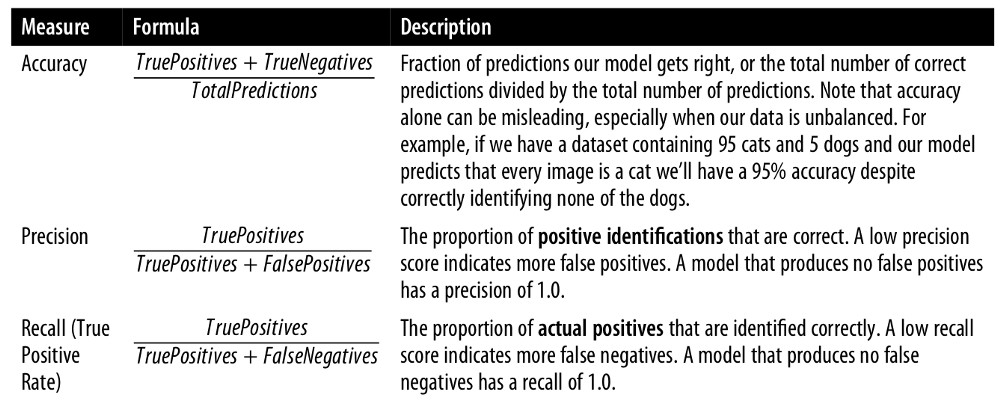Figure 5. Accuracy measures

scikit-learn has built in functions that we can use for this. The following function will help with this:

``````from sklearn.metrics import recall_score
from sklearn.metrics import precision_score
from sklearn.metrics import accuracy_score

def evaluate_model(predictions, actual):
return pd.DataFrame({
"Measure": ["Accuracy", "Precision", "Recall"],
"Score": [accuracy_score(actual, predictions),
precision_score(actual, predictions),
recall_score(actual, predictions)]
})``````

We can evaluate our model by running the following code:

``````predictions = classifier.predict(df_test_under[columns])
y_test = df_test_under["label"]

evaluate_model(predictions, y_test)``````
Table 5. Results
Measure Score

Accuracy

0.9636844377293331

Precision

0.9607506702412869

Recall

0.9668681200086338

Our model has done pretty well at predicting whether there is likely to be a co-authorship between a pair of authors. It scores above 96% on all of the evaluation metrics.

## Next Steps

We’ve already got a good model, but can we do better?

Perhaps we could try using a different algorithm, or even the same algorithm with different hyper-parameters. Or maybe we can add more features based on the results of other algorithms.

If you have any ideas or questions, please create an issue or PR on the neo4j-examples/link-prediction GitHub repository.